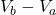## Points a and b lie in a region where the y-component of the electric field is Ey=α+β/y2. The constants in this expression have the values α

Question

Points a and b lie in a region where the y-component of the electric field is Ey=α+β/y2. The constants in this expression have the values α = 600 N/C and β = 5.00 N⋅m2/C. Points a and b are on the y-axis. .

Point a is at y = 2.00 cm and point b is at y = 3.00 cm. What is the potential difference Va−Vb between these two points.?

in progress 0
5 months 2021-08-25T11:31:19+00:00 1 Answers 17 views 0

V_{a} – V_{b} = 89.3

Explanation:

The electric potential is defined by= – ∫ E .ds

In this case the electric field is in the direction and the points (ds) are also in the direction and therefore the angle is zero and the scalar product is reduced to the algebraic product.

V_{b} – V_{a} = – ∫ E ds

We substitute

V_{b} – V_{a} = – ∫ (α + β/ y²) dy

We integrate

V_{b} – V_{a} = – α y + β / y

We evaluate between the lower limit A  2 cm = 0.02 m and the upper limit B 3 cm = 0.03 m

V_{b} – V_{a} = – α (0.03 – 0.02) + β (1 / 0.03 – 1 / 0.02)

V_{b} – V_{a} = – 600 0.01 + 5 (-16.67) = -6 – 83.33

V_{b} – V_{a} = – 89.3 V

As they ask us the reverse case

V_{b} – V_{a} = – V_{b} – V_{a}

V_{a} – V_{b} = 89.3